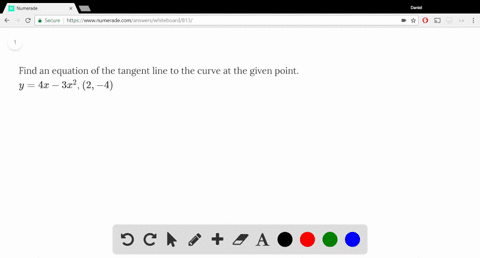Enroll in one of our FREE online STEM summer camps. Space is limited so join now!View Summer Courses### Find an equation of the tangent line to the curve…

03:17

Need more help? Fill out this quick form to get professional live tutoring.

Get live tutoring
Problem 4

(a) Find the slope of the tangent line to the curve $y = x - x^3$ at the point $(1, 0)$
(i) using Definition 1 (ii) using Equation 2

(b) Find an equation of the tangent line in part (a).

(c) Graph the curve and the tangent line in successively smaller viewing rectangles centered at $(1, 0)$ until the curve and the line appear to coincide.

a) (i) -2
(ii) -2
b) $y=-2 x+2$
c) graph unavailable

## Discussion

You must be signed in to discuss.

## Video Transcript

this problem Number four of this to a calculus eight edition section two point seven. I find the slope of the tangent line to the curve. Why equals X minus X cubed or at the point one zero, using definition one and using the equation to definition one an equation to our shooting here for your reference. And we begin with the finish in one, the slope can be found by calculating the limit as experts, eh? Where in this problem is one, since that is the point of interest, that's the exploits of the point of interest. One zero. The X is a protein one of the function f of X, which is X minus X cubed, minus your function Evaluated, eh? So at AIDS one one minus one cube, well, that's just a zero. So the function remains Are the function is X minus X cubed minus the function value added a one zero on the right abi X minus one or mix minutes, eh? If we simplify this function in the numerator, we can factor out next and we're left with that one minus X squared. Well, go ahead and also factored this term because it's the difference affairs of squares into one minute. Sex one plus six. Um, and the numerator denominator is explain this one. We can go ahead and cancel this term and this term exhibit They're not exactly the same. There's going to be a leftover negative one. Um, after it is cancelled. Um, and then the next step will be to plug in er the value one here, so the answer would be a negative one times one, which is the sex multiplied by one plus one or two. And I can see it's an answer. Negative, too, for the soup. And that's using definition one. Using equation, too. We have a limited h of purchase, a serial of the function evaluated a A plus h where is one? So we're going to be in putting one plus age wherever there's an X. So it's one plus each, minus the quantity one plus h cubed. And we're going to be subjecting FAA, which we saw in this previous part that that term is zero and we're dividing by h. Okay, So in order to simplify, we're going to expand the generator one plus age minus on this term when it is when it is expanded or beyond. Plus, each times one plus X squared will That's one place to age plus eight squared. And if we combined those two in the next champ, the numerator will be ah, rim plus age minus one plus to age plus eight. Squared class, Age plus two h squared was h cubed all over h here in the numerator the term one and negative one Counsel, Um, and simplifying down here and combining like terms. We have a tch minus two age. That's negative. H ah, minus another age. Think of two each. That's the first term. And then we're left over with any squared in a two h squared. That's three. It's squared with the negative in front negative three x squared on and then finally left over with negative h cubed over each. Ah, now we plug in or we cancel out one h her turn here we're left with it's squared and then we plug in. H is zero. That cancels out these Final two terms, and we're left over with an answer negative to which agrees with the answer for definition. One for part B. Renee's a slope negative too. And we're going to write an equation of attention line with the point one zero. So we have a point and we have a slope. We're in his point slope form. Why I'm on this white one equals M times x twenty six one where the point is a war memento that zero to the point. It's ones here. A superstate of two and X is one. So our function is actually going to be y equals native to x Ah, plus two. And this is the equation of the tension line according to the information given a part of B finally in part see graft, the curve and the tangent line and successively smaller, reeling rectangles centered at one zero until the curve and the line appear to coincide. So we take the function, explain its execute, and we're also going to plot the tension line Negative too explicit. Two. And here we see an animation ah, zooming into the point one zero. So the orange function that is exponents x cubed this purple function. The linear function is the tension line Negative two x plus two and as we see here, it is tangent to the curve at one zero And as we successively Suman into the point one zero, the no attention lane and the curve coincide and become indistinguishable. So this is the purpose of this exercise in party. We have shown that this the line is indeed tangent at one zero and that is reason closer. The ah functions are index in distinguishing.